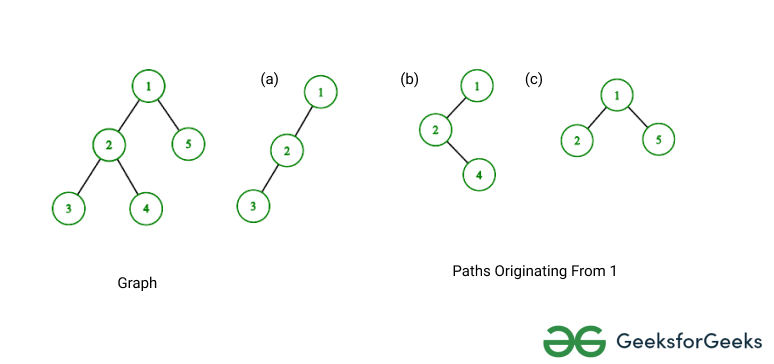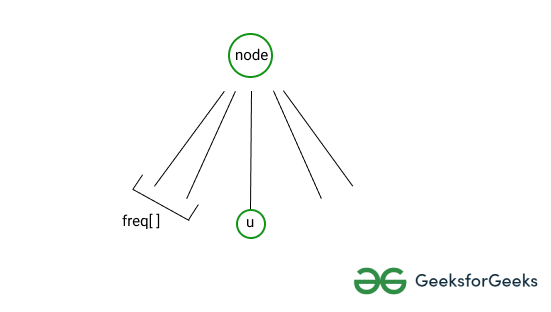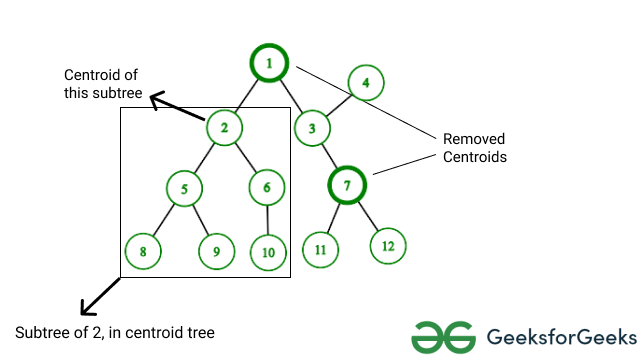# Number of K-length paths in a Tree

• Difficulty Level : Hard
• Last Updated : 10 Jan, 2022

Given a tree of N nodes and an integer K, the task is to find the total number of paths having length K.

Examples:

Input: N = 5, K = 2
tree =           1
/  \
2    5
/  \
3    4
Output: 4
Explanation: The paths having length 2 are
1 – 2 – 3, 1 – 2 – 4, 2 – 1 – 5, 3 – 2 – 4

Input: N = 2, K = 2
tree =       1
/
2
Output: 0
Explanation: There is no path in the tree having length 2.

Intuition: The main idea is to find the K-length paths from each node and add them.

1. Find the number of K-length paths ‘originating’ from a given node ‘node’. ‘Originating’ here means, ‘node’ will have the smallest depth among all the nodes in the path. For example, 2-length paths originating from 1 are shown in the below diagram.
2. Sum above value for all the nodes and it will be the required answer.Naive Approach: To compute the K-length paths originating from ‘node’ two DFS are used. Say this entire process is: paths_originating_from(node)

1. Suppose ‘node’ has multiple children and currently child ‘u’ is being processed.
2. For all the previous children, the frequency of nodes at a particular depth has been calculated. More formally, freq[d] gives the number of nodes at depth ‘d’ when only children of ‘node’ before ‘u’ have been processed.
3. If there is a node ‘x’ at depth ‘d’, number of K length paths originating from ‘node’ and passing through ‘x’ will be freq[K – d].
4. The first DFSF would contribute to the final answer, and the second DFS would update the freq[] array for future use.
5. Sum-up ‘paths_originating_from(node)’ for all nodes of the tree, this will be the required answer.

See the image below to understand the 2nd point better.Below is the implementation of the above approach.

## C++

 `// C++ code to implement above approach``#include ``using` `namespace` `std;`` ` `int` `mx_depth = 0, ans = 0;``int` `N, K;``vector<``int``> freq;``vector > g;`` ` `// This dfs is responsible for calculating ans ``// and updating freq vector``void` `dfs(``int` `node, ``int` `par, ``int` `depth, ``         ``bool` `contri)``{``    ``if` `(depth > K)``        ``return``;``    ``mx_depth = max(mx_depth, depth);`` ` `    ``if` `(contri) {``        ``ans += freq[K - depth];``    ``}``    ``else` `{``        ``freq[depth]++;``    ``}`` ` `    ``for` `(``auto` `nebr : g[node]) {``        ``if` `(nebr != par) {``            ``dfs(nebr, node, depth + 1, ``                ``contri);``        ``}``    ``}``}`` ` `// Function to calculate K length paths ``// originating from node``void` `paths_originating_from(``int` `node, ``                            ``int` `par)``{``    ``mx_depth = 0;``    ``freq = 1;`` ` `    ``// For every not-removed nebr, ``    ``// calculate its contribution, ``    ``// then update freq vector for it``    ``for` `(``auto` `nebr : g[node]) {``        ``if` `(nebr != par) {``            ``dfs(nebr, node, 1, ``true``);``            ``dfs(nebr, node, 1, ``false``);``        ``}``    ``}`` ` `    ``// Re-initialize freq vector``    ``for` `(``int` `i = 0; i <= mx_depth; ++i) {``        ``freq[i] = 0;``    ``}`` ` `    ``// Repeat the same for children``    ``for` `(``auto` `nebr : g[node]) {``        ``if` `(nebr != par) {``            ``paths_originating_from(nebr, ``                                   ``node);``        ``}``    ``}``}`` ` `// Utility method to add edges to tree``void` `edge(``int` `a, ``int` `b)``{``    ``a--;``    ``b--;``    ``g[a].push_back(b);``    ``g[b].push_back(a);``}`` ` `// Driver code``int` `main()``{``    ``N = 5, K = 2;``    ``freq = vector<``int``>(N);``    ``g = vector >(N);`` ` `    ``edge(1, 2);``    ``edge(1, 5);``    ``edge(2, 3);``    ``edge(2, 4);``   ` `    ``paths_originating_from(0, -1);``    ``cout << ans << endl;``}`

Output

```4
```

Time Complexity: O(N * H) where H is the height of the tree which can be N at max
Auxiliary Space: O(N)

Efficient Approach: This approach is based on the concept of Centroid Decomposition. The steps are as follows:

1. Find the centroid of current tree T.
2. All ‘not-removed’ nodes reachable from T belong to its sub-tree. Call paths_originating_from(T), then mark T as ‘removed’.
3. Repeat the above process for all ‘not-removed’ neighbors of T.

The following figure shows a tree with current centroid and its sub-tree. Note that nodes with thick borders have already been selected as centroids previously and do not belong to the sub-tree of the current centroid.Below is the implementation of the above approach.

## C++

 `// C++ code to implement above approach``#include ``using` `namespace` `std;`` ` `// Struct for centroid decomposition``struct` `CD {``    ``// 1. mx_depth will be used to store ``    ``// the height of a node``    ``// 2. g[] is adjacency list for tree``    ``// 3. freq[] stores frequency of nodes ``    ``// at particular height, it is maintained ``    ``// for children of a node``    ``int` `n, k, mx_depth, ans;``    ``vector<``bool``> removed;``    ``vector<``int``> size, freq;``    ``vector > g;`` ` `    ``// Constructor for struct``    ``CD(``int` `n1, ``int` `k1)``    ``{``        ``n = n1;``        ``k = k1;``        ``ans = mx_depth = 0;`` ` `        ``g.resize(n);``        ``size.resize(n);``        ``freq.resize(n);``        ``removed.assign(n, ``false``);``    ``}`` ` `    ``// Utility method to add edges to tree``    ``void` `edge(``int` `u, ``int` `v)``    ``{``        ``u--;``        ``v--;``        ``g[u].push_back(v);``        ``g[v].push_back(u);``    ``}`` ` `    ``// Finds size of a subtree, ``    ``// ignoring removed nodes in the way``    ``int` `get_size(``int` `node, ``int` `par)``    ``{``        ``if` `(removed[node])``            ``return` `0;``        ``size[node] = 1;`` ` `        ``for` `(``auto` `nebr : g[node]) {``            ``if` `(nebr != par) {``                ``size[node] += get_size(nebr, ``                                       ``node);``            ``}``        ``}`` ` `        ``return` `size[node];``    ``}`` ` `    ``// Calculates centroid of a subtree ``    ``// of 'node' of size 'sz'``    ``int` `get_centroid(``int` `node, ``int` `par, ``                     ``int` `sz)``    ``{``        ``for` `(``auto` `nebr : g[node]) {``            ``if` `(nebr != par && !removed[nebr]``                ``&& size[nebr] > sz / 2) {``                ``return` `get_centroid(nebr, ``                                    ``node, sz);``            ``}``        ``}``        ``return` `node;``    ``}`` ` `    ``// Decompose the tree ``    ``// into various centroids``    ``void` `decompose(``int` `node, ``int` `par)``    ``{``        ``get_size(node, -1);`` ` `        ``// c is centroid of subtree 'node'``        ``int` `c = get_centroid(node, par, ``                             ``size[node]);`` ` `        ``// Find paths_originating_from 'c'``        ``paths_originating_from(c);`` ` `        ``// Mark this centroid as removed``        ``removed = ``true``;`` ` `        ``// Find other centroids``        ``for` `(``auto` `nebr : g) {``            ``if` `(!removed[nebr]) {``                ``decompose(nebr, c);``            ``}``        ``}``    ``}`` ` `    ``// This dfs is responsible for ``    ``// calculating ans and ``    ``// updating freq vector``    ``void` `dfs(``int` `node, ``int` `par, ``int` `depth,``             ``bool` `contri)``    ``{``        ``if` `(depth > k)``            ``return``;``        ``mx_depth = max(mx_depth, depth);`` ` `        ``if` `(contri) {``            ``ans += freq[k - depth];``        ``}``        ``else` `{``            ``freq[depth]++;``        ``}`` ` `        ``for` `(``auto` `nebr : g[node]) {``            ``if` `(nebr != par && ``                ``!removed[nebr]) {``                ``dfs(nebr, node, ``                    ``depth + 1, contri);``            ``}``        ``}``    ``}`` ` `    ``// Function to find K-length paths``    ``// originating from node``    ``void` `paths_originating_from(``int` `node)``    ``{``        ``mx_depth = 0;``        ``freq = 1;`` ` `        ``// For every not-removed nebr, ``        ``// calculate its contribution, ``        ``// then update freq vector for it``        ``for` `(``auto` `nebr : g[node]) {``            ``if` `(!removed[nebr]) {``                ``dfs(nebr, node, 1, ``true``);``                ``dfs(nebr, node, 1, ``false``);``            ``}``        ``}``         ` `        ``// Re-initialize freq vector``        ``for` `(``int` `i = 0; i <= mx_depth; ++i) {``            ``freq[i] = 0;``        ``}``    ``}``};`` ` `// Driver code``int` `main()``{``    ``int` `N = 5, K = 2;`` ` `    ``CD cd_s(N, K);``    ``cd_s.edge(1, 2);``    ``cd_s.edge(1, 5);``    ``cd_s.edge(2, 3);``    ``cd_s.edge(2, 4);`` ` `    ``cd_s.decompose(0, -1);``    ``cout << cd_s.ans;``    ``return` `0;``}`

Output

`4`

Time Complexity: O(N * log(N)) where log N is the height of the tree
Auxiliary Space: O(N)

My Personal Notes arrow_drop_up